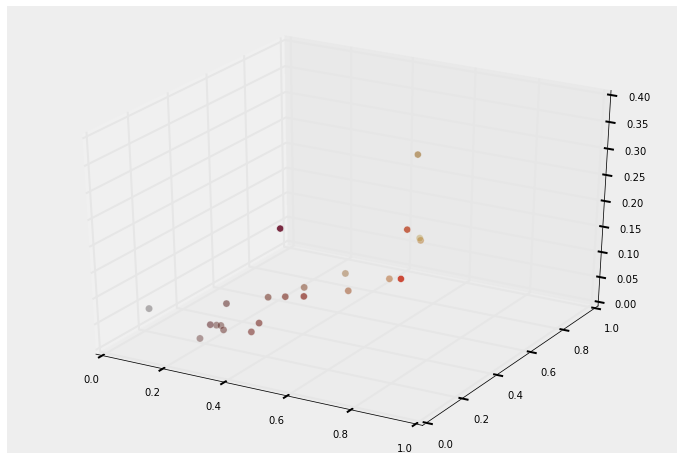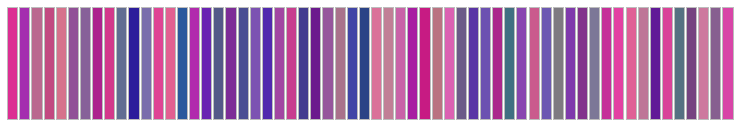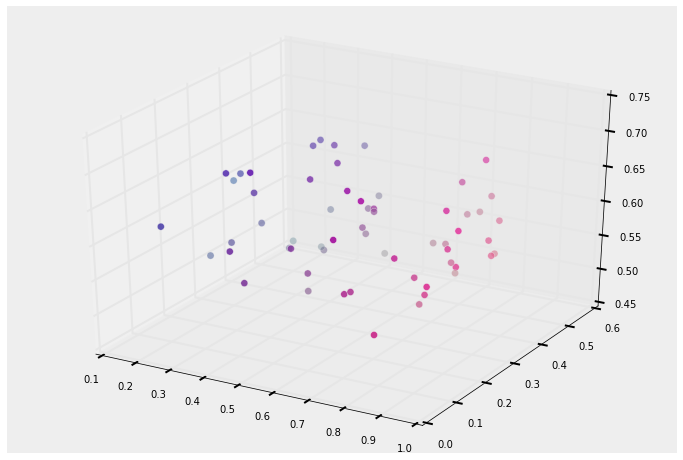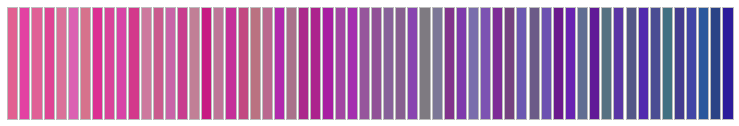## Using PCA to sort high dimensional objects¶

• Recall that PCA maximizes information retained when we project our data to lower dimensions.

• Even when projecting our dataset down to 1 dimension, PCA will capture as much information as possible from the original dataset.

• What's unique about 1 dimension data? Well it's an array, and it can be sorted!

• So if we have objects that can be represented as a high-dimnesional vector, we have an oppurtunity to endow an "order" to them somehow (now you can't see it, but I am air-quoting "order"). This order has nothing to do with one object being "better", or "larger" (again air quotes) than another object, but instead has to do with an ordering that minimizes information lost. What does that mean?

• Let's try this with colors!

• Colors can exist in 3 dimensions, and the dimensions are R (red), G (green) and B (blue).

• This example actually came from a problem I had. I wanted to sort my books my color, like:I took a photo of a subset of my book collection, sampled what color they were using Gimp, and recorded the colour in rgb.

Sorting them was going to be inpractical: it would take time - plus it's actually really hard to decide on an order for colors. Example:

In [ ]:
%pylab inline
from mpl_toolkits.mplot3d import Axes3D
figsize(12,8)

colours = np.array([[111,  28,  21],
[ 27,  17,  20],
[ 79,  23,  17],
[185, 125,  50],
[155,  76,  32],
[ 82,  24,  17],
[127,  63,  33],
[193,  91,  63],
[176,  97,  36],
[ 79,  15,  19],
[176, 140,  47],
[203,  65,  46],
[174, 139,  87],
[138,  44,  34],
[144,  91,  36],
[ 86,  21,  16],
[123,  44,  32],
[109,  44,  30],
[ 84,  29,  27],
[121,  42,  65],
[ 70,  15,  11],
[107,  24,  17]])/255.0

# I've normalized the colours between 0 and 1



This a the color list of a subset of library that I pulled from a photo. There are 22 book colours here, and I've normalized the dimensions to be between 0 and 1.

In [ ]:
def display_colours(colours):
figsize(13,2)
N = colours.shape
for i,c in enumerate(colours):
ax = subplot(1,N,(i+1)%N, axisbg=c)
ax.get_xaxis().set_visible(False)
ax.get_yaxis().set_visible(False)

plt.show()


This is a little helper function that will display a list of colours passed in.

In :
display_colours(colours)

Populating the interactive namespace from numpy and matplotlib(22, 3)


You can probably do a pretty good job of sorting these, though there are some odd ones:

• where does that purple on go?
• and this orange one?

But it is pretty time consuming even for only 22 colours here, and the process is very subjective. Likely your friend will have a very different ordering. We'd like something objective, and that scales to potentially hundreds or thousands of colors.

Let's next visualize these colours in 3-space:

In :
figsize(12,8)
fig = plt.figure()

ax.scatter(colours[:,0], colours[:,1], colours[:,2],
c =colours, s=50 )
plt.xlim(0,1)
plt.ylim(0,1)
plt.show()What we'd like to do

1. Find a 1-dimensional representation of each book's color, that way it can be sorted.
2. Determine some ordering. Consider the following made-up example: An ordering that sorts based on the amount of blue in a set of books that are only mostly green and red book would be very poor, as the human eye would have difficult time seeing the amount of blue vary over the ordering. Instead, what we want is colors that are distant in 3-D to be very distant in 1-D too, similarly for close colours: colours close in 3-space, and appear visally similar, should be close in 1 space too . Thus, I would like an ordering that maximizes the amount of variation when we project to 1-D.

This is exactly what PCA does. So let's do that:

In :
from sklearn.decomposition import PCA
pca = PCA(n_components=1)
# I'm setting n_components to 1 as we are projecting down to only 1 dimension

In :
one_d_colours = pca.fit_transform(colours)[:,0]
print one_d_colours.shape
print one_d_colours

(22,)
[-0.1251 -0.3963 -0.2339  0.356   0.1311 -0.2228  0.019   0.3082  0.2474
-0.2516  0.3639  0.2567  0.3946  0.0051  0.14   -0.2195 -0.0403 -0.0827
-0.1949 -0.0188 -0.2855 -0.1504]

In :
ix = np.argsort(one_d_colours)
#what I want is the sorted order of elements in 1-dimension, and I'll use that in
# ordering the colours.

In :
display_colours(colours[ix])### Let's try (sorta) random colors¶

So that worked well, but let's try some more diverse colors. Below I'll generate 60 random colours from a subset of the unit cube.

In :
N = 60
colours = np.random.uniform([0.15,0.1,0.5], [.9,0.5,0.7], size=(N,3))

In :
#here are the colours, unsorted
display_colours(colours)In :
figsize(12,8)
fig = plt.figure()
ax.scatter(colours[:,0], colours[:,1], colours[:,2],
c =colours, s=50 )
plt.show()In :
one_d_colours = pca.fit_transform(colours)[:,0]

ix = np.argsort(one_d_colours)

display_colours(colours[ix])This looks pretty good to me, I'd grade it an 80%. There are some oddities, but considering what is means to "sort colors", I'd say it did a pretty decent job.

## Conclusion¶

Recall that this method of sorting is completely unsupervised: there is no "better" or "larger" colour. PCA is finding the line in 3-space that maximizes the data variance in 1-space. This line also happens to be what we wanted: we wanted different colors in 3-space to be maximally different in 1-space too.

I chose rgb space. Though this way arbitrary. You could also use other color schemes: HSV, HSL, or CMYK. HSV might even produce better results, as it was designed to be more intuitive and perceptually relevant than RGB. Interestingly, CMYK colors would live in 4 dimensions originally, not 3 like RGB.

On sorting colors: There is acutally a better way to sort colours using the travelling salesman problem, but I'll explain that in another lecture.

In :


In :


In [ ]: